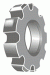I have forgotten

•http://facebook.com/
•https://www.google.com/accounts/o8/id
•https://me.yahoo.com

# Equation editor plugin: image quality7 Sep 15, 9:50AM
(2 replies)
Equation editor plugin: image quality
Hello,

Is there a way to improve the resolution of the image rendered by the CodeCogs engine?

Please see the image here (CKEditor content was exported to PDF):

Is there a way to control the resolution of this image? The current quality is not acceptable for our workflow.

Thanks, Prabal.7 Sep 15, 1:14PM
There are two routes you might want to consider:

1) Generate a Hi Res Gif Images

Starting with a standard equation request, i.e.
`http://latex.codecogs.com/gif.latex?1+sin(x^2)`
which generate this: http://latex.codecogs.com/gif.latex?1+sin(x^2)

Add "\dpi{300}" (say) to the start of the line to get a hi-res images, i.e.
`http://latex.codecogs.com/gif.latex?\dpi{300}1+sin(x^2)`
which generate this: http://latex.codecogs.com/gif.latex?\dpi{300}1+sin(x^2)

2) Generate an svg or pdf image

A vector image can then be embedded into your output document. This just requires a small change to the url, i.e.
`http://latex.codecogs.com/svg.latex?1+sin(x^2)`
which generate this: http://latex.codecogs.com/svg.latex?1+sin(x^2)

or
`http://latex.codecogs.com/pdf.latex?1+sin(x^2)`
which generate this: http://latex.codecogs.com/pdf.latex?1+sin(x^2)7 Sep 15, 1:41PM
Thanks! I tried both and here are my observations:

1. Increasing the resolution did create a higher quality image, but also resulted in a larger dimension. Is there a way to constrain the dimension of the image?